# Introduction to Pseudocode

Nov. 28, 2011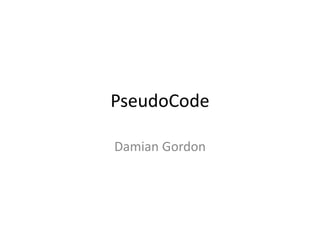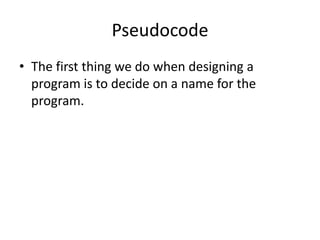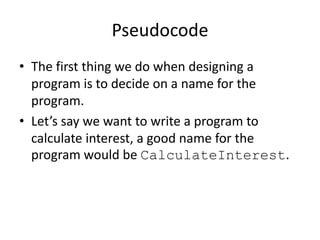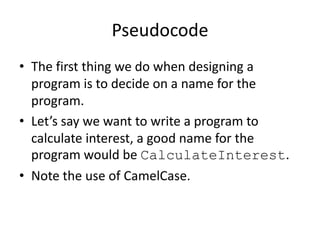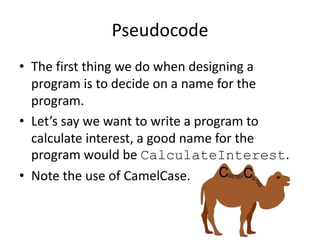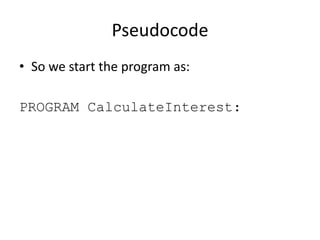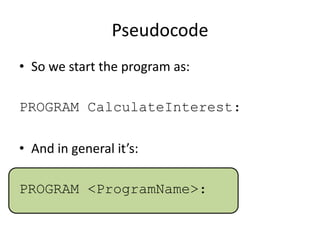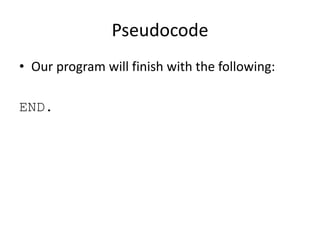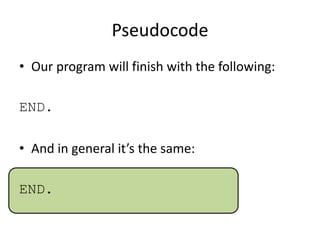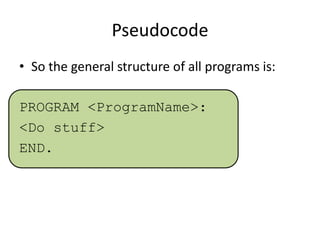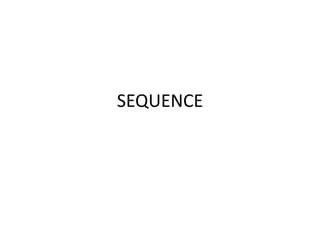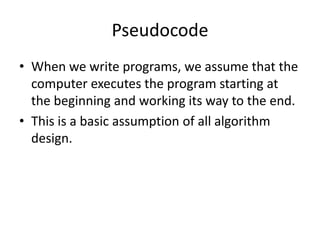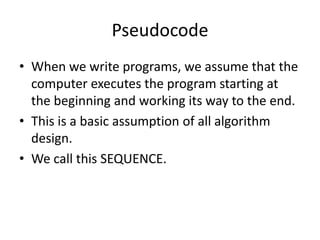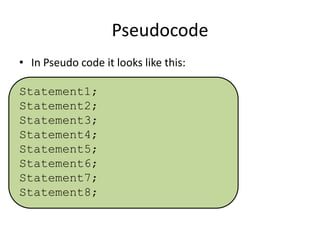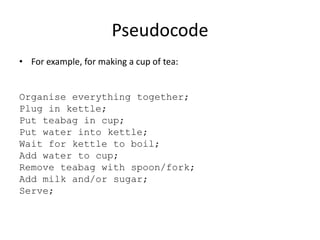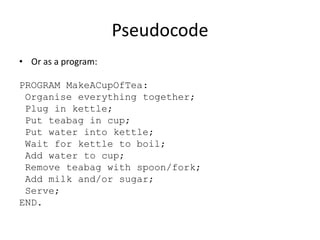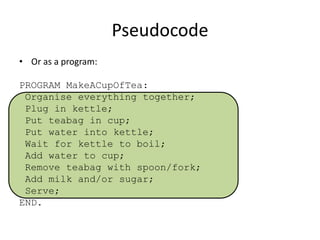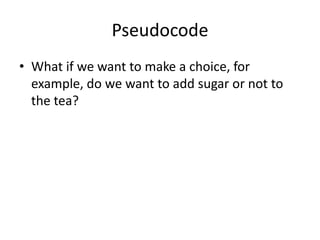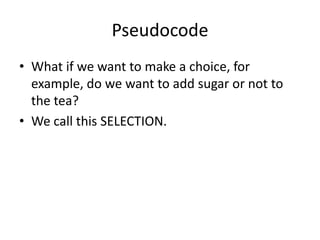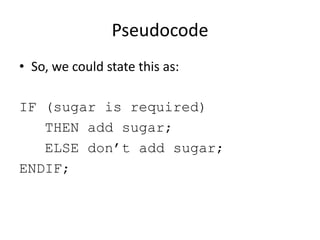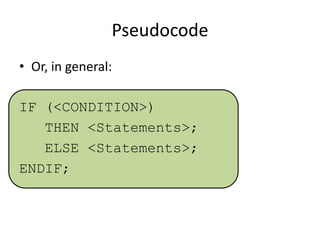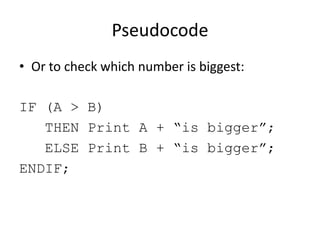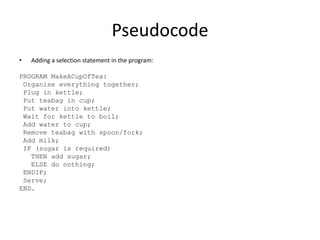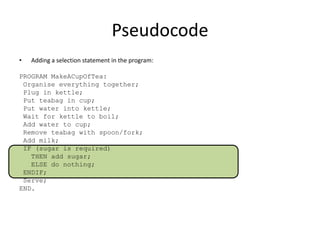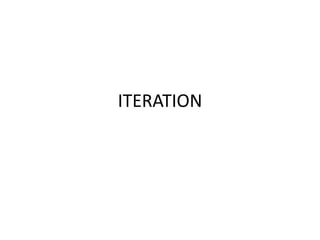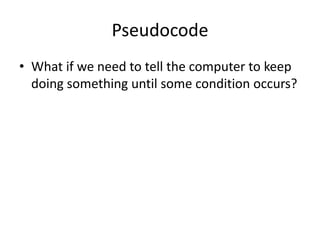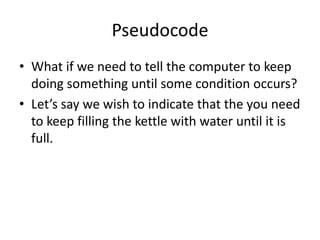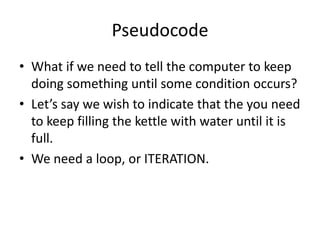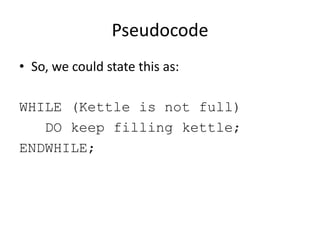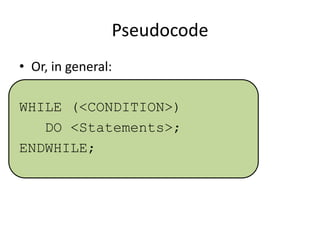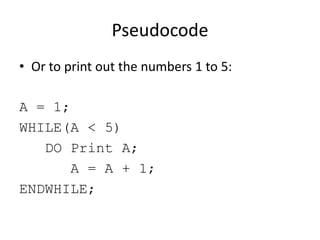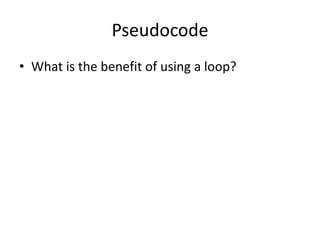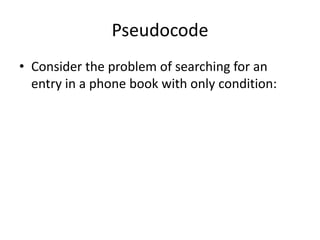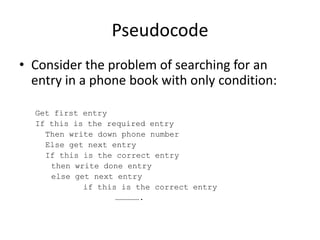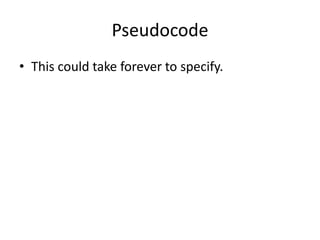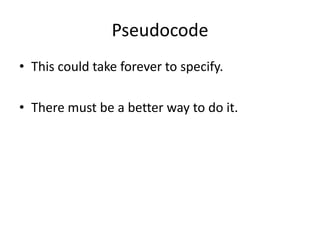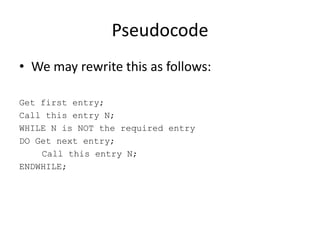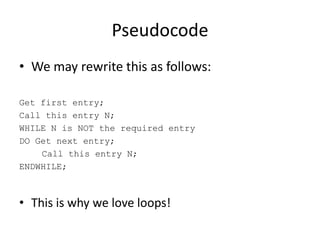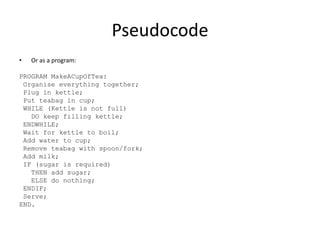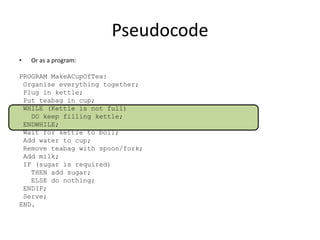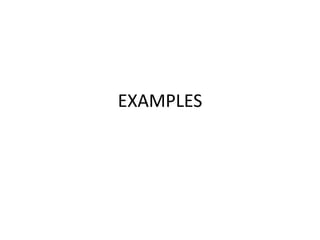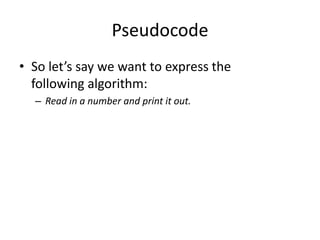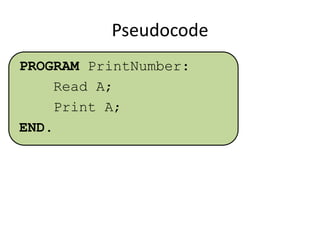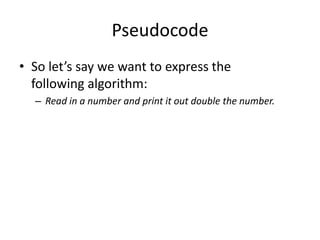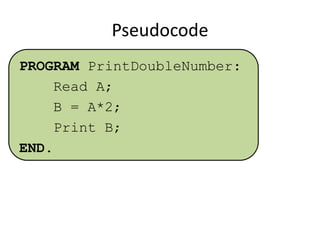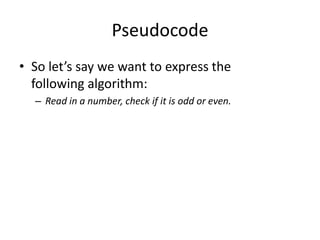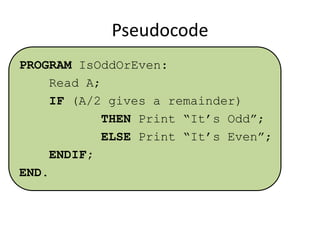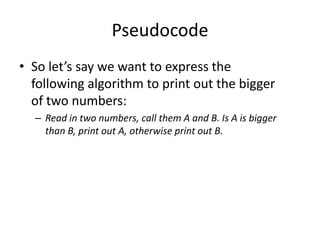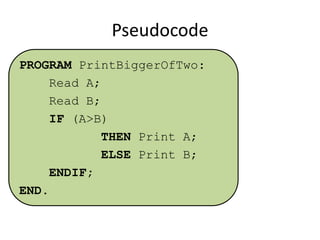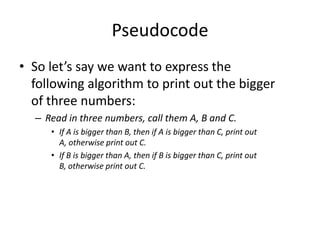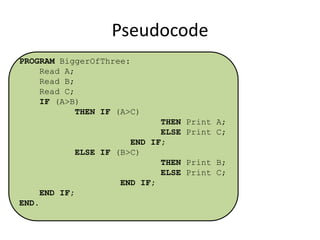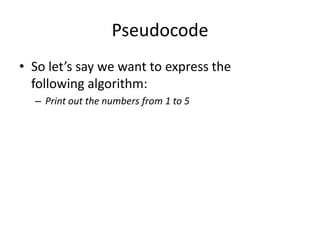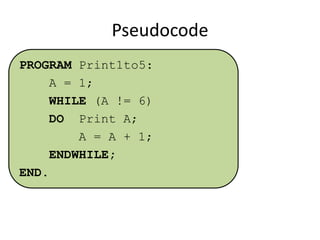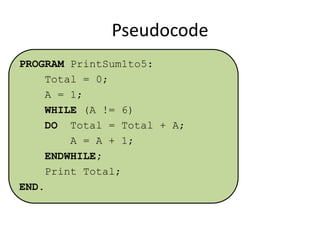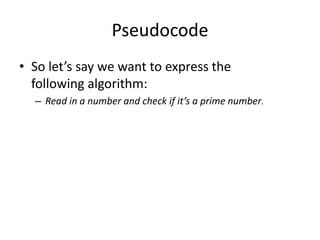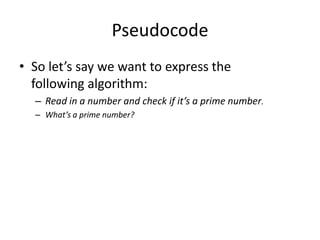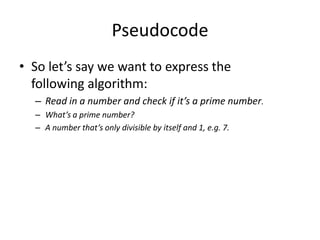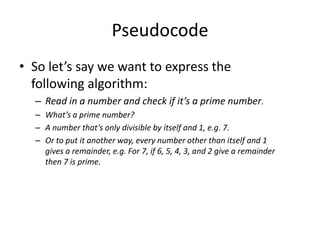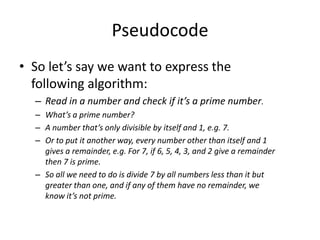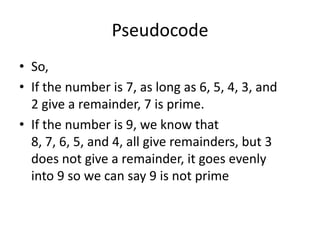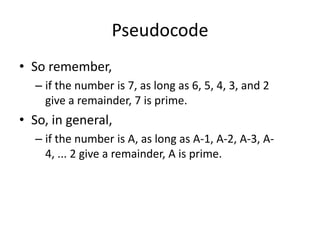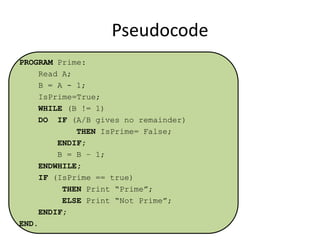1 of 64

### Introduction to Pseudocode

• 1. PseudoCode Damian Gordon
• 2. Pseudocode • The first thing we do when designing a program is to decide on a name for the program.
• 3. Pseudocode • The first thing we do when designing a program is to decide on a name for the program. • Let’s say we want to write a program to calculate interest, a good name for the program would be CalculateInterest.
• 4. Pseudocode • The first thing we do when designing a program is to decide on a name for the program. • Let’s say we want to write a program to calculate interest, a good name for the program would be CalculateInterest. • Note the use of CamelCase.
• 5. Pseudocode • The first thing we do when designing a program is to decide on a name for the program. • Let’s say we want to write a program to calculate interest, a good name for the program would be CalculateInterest. • Note the use of CamelCase.
• 6. Pseudocode • So we start the program as: PROGRAM CalculateInterest:
• 7. Pseudocode • So we start the program as: PROGRAM CalculateInterest: • And in general it’s: PROGRAM <ProgramName>:
• 8. Pseudocode • Our program will finish with the following: END.
• 9. Pseudocode • Our program will finish with the following: END. • And in general it’s the same: END.
• 10. Pseudocode • So the general structure of all programs is: PROGRAM <ProgramName>: <Do stuff> END.
• 11. SEQUENCE
• 12. Pseudocode • When we write programs, we assume that the computer executes the program starting at the beginning and working its way to the end. • This is a basic assumption of all algorithm design.
• 13. Pseudocode • When we write programs, we assume that the computer executes the program starting at the beginning and working its way to the end. • This is a basic assumption of all algorithm design. • We call this SEQUENCE.
• 14. Pseudocode • In Pseudo code it looks like this: Statement1; Statement2; Statement3; Statement4; Statement5; Statement6; Statement7; Statement8;
• 15. Pseudocode • For example, for making a cup of tea: Organise everything together; Plug in kettle; Put teabag in cup; Put water into kettle; Wait for kettle to boil; Add water to cup; Remove teabag with spoon/fork; Add milk and/or sugar; Serve;
• 16. Pseudocode • Or as a program: PROGRAM MakeACupOfTea: Organise everything together; Plug in kettle; Put teabag in cup; Put water into kettle; Wait for kettle to boil; Add water to cup; Remove teabag with spoon/fork; Add milk and/or sugar; Serve; END.
• 17. Pseudocode • Or as a program: PROGRAM MakeACupOfTea: Organise everything together; Plug in kettle; Put teabag in cup; Put water into kettle; Wait for kettle to boil; Add water to cup; Remove teabag with spoon/fork; Add milk and/or sugar; Serve; END.
• 18. SELECTION
• 19. Pseudocode • What if we want to make a choice, for example, do we want to add sugar or not to the tea?
• 20. Pseudocode • What if we want to make a choice, for example, do we want to add sugar or not to the tea? • We call this SELECTION.
• 21. Pseudocode • So, we could state this as: IF (sugar is required) THEN add sugar; ELSE don’t add sugar; ENDIF;
• 22. Pseudocode • Or, in general: IF (<CONDITION>) THEN <Statements>; ELSE <Statements>; ENDIF;
• 23. Pseudocode • Or to check which number is biggest: IF (A > B) THEN Print A + “is bigger”; ELSE Print B + “is bigger”; ENDIF;
• 24. Pseudocode • Adding a selection statement in the program: PROGRAM MakeACupOfTea: Organise everything together; Plug in kettle; Put teabag in cup; Put water into kettle; Wait for kettle to boil; Add water to cup; Remove teabag with spoon/fork; Add milk; IF (sugar is required) THEN add sugar; ELSE do nothing; ENDIF; Serve; END.
• 25. Pseudocode • Adding a selection statement in the program: PROGRAM MakeACupOfTea: Organise everything together; Plug in kettle; Put teabag in cup; Put water into kettle; Wait for kettle to boil; Add water to cup; Remove teabag with spoon/fork; Add milk; IF (sugar is required) THEN add sugar; ELSE do nothing; ENDIF; Serve; END.
• 26. ITERATION
• 27. Pseudocode • What if we need to tell the computer to keep doing something until some condition occurs?
• 28. Pseudocode • What if we need to tell the computer to keep doing something until some condition occurs? • Let’s say we wish to indicate that the you need to keep filling the kettle with water until it is full.
• 29. Pseudocode • What if we need to tell the computer to keep doing something until some condition occurs? • Let’s say we wish to indicate that the you need to keep filling the kettle with water until it is full. • We need a loop, or ITERATION.
• 30. Pseudocode • So, we could state this as: WHILE (Kettle is not full) DO keep filling kettle; ENDWHILE;
• 31. Pseudocode • Or, in general: WHILE (<CONDITION>) DO <Statements>; ENDWHILE;
• 32. Pseudocode • Or to print out the numbers 1 to 5: A = 1; WHILE(A < 5) DO Print A; A = A + 1; ENDWHILE;
• 33. Pseudocode • What is the benefit of using a loop?
• 34. Pseudocode • Consider the problem of searching for an entry in a phone book with only condition:
• 35. Pseudocode • Consider the problem of searching for an entry in a phone book with only condition: Get first entry If this is the required entry Then write down phone number Else get next entry If this is the correct entry then write done entry else get next entry if this is the correct entry …………….
• 36. Pseudocode • This could take forever to specify.
• 37. Pseudocode • This could take forever to specify. • There must be a better way to do it.
• 38. Pseudocode • We may rewrite this as follows: Get first entry; Call this entry N; WHILE N is NOT the required entry DO Get next entry; Call this entry N; ENDWHILE;
• 39. Pseudocode • We may rewrite this as follows: Get first entry; Call this entry N; WHILE N is NOT the required entry DO Get next entry; Call this entry N; ENDWHILE; • This is why we love loops!
• 40. Pseudocode • Or as a program: PROGRAM MakeACupOfTea: Organise everything together; Plug in kettle; Put teabag in cup; WHILE (Kettle is not full) DO keep filling kettle; ENDWHILE; Wait for kettle to boil; Add water to cup; Remove teabag with spoon/fork; Add milk; IF (sugar is required) THEN add sugar; ELSE do nothing; ENDIF; Serve; END.
• 41. Pseudocode • Or as a program: PROGRAM MakeACupOfTea: Organise everything together; Plug in kettle; Put teabag in cup; WHILE (Kettle is not full) DO keep filling kettle; ENDWHILE; Wait for kettle to boil; Add water to cup; Remove teabag with spoon/fork; Add milk; IF (sugar is required) THEN add sugar; ELSE do nothing; ENDIF; Serve; END.
• 42. EXAMPLES
• 43. Pseudocode • So let’s say we want to express the following algorithm: – Read in a number and print it out.
• 44. Pseudocode PROGRAM PrintNumber: Read A; Print A; END.
• 45. Pseudocode • So let’s say we want to express the following algorithm: – Read in a number and print it out double the number.
• 46. Pseudocode PROGRAM PrintDoubleNumber: Read A; B = A*2; Print B; END.
• 47. Pseudocode • So let’s say we want to express the following algorithm: – Read in a number, check if it is odd or even.
• 48. Pseudocode PROGRAM IsOddOrEven: Read A; IF (A/2 gives a remainder) THEN Print “It’s Odd”; ELSE Print “It’s Even”; ENDIF; END.
• 49. Pseudocode • So let’s say we want to express the following algorithm to print out the bigger of two numbers: – Read in two numbers, call them A and B. Is A is bigger than B, print out A, otherwise print out B.
• 50. Pseudocode PROGRAM PrintBiggerOfTwo: Read A; Read B; IF (A>B) THEN Print A; ELSE Print B; ENDIF; END.
• 51. Pseudocode • So let’s say we want to express the following algorithm to print out the bigger of three numbers: – Read in three numbers, call them A, B and C. • If A is bigger than B, then if A is bigger than C, print out A, otherwise print out C. • If B is bigger than A, then if B is bigger than C, print out B, otherwise print out C.
• 52. Pseudocode PROGRAM BiggerOfThree: Read A; Read B; Read C; IF (A>B) THEN IF (A>C) THEN Print A; ELSE Print C; END IF; ELSE IF (B>C) THEN Print B; ELSE Print C; END IF; END IF; END.
• 53. Pseudocode • So let’s say we want to express the following algorithm: – Print out the numbers from 1 to 5
• 54. Pseudocode PROGRAM Print1to5: A = 1; WHILE (A != 6) DO Print A; A = A + 1; ENDWHILE; END.
• 55. Pseudocode • So let’s say we want to express the following algorithm: – Add up the numbers 1 to 5 and print out the result
• 56. Pseudocode PROGRAM PrintSum1to5: Total = 0; A = 1; WHILE (A != 6) DO Total = Total + A; A = A + 1; ENDWHILE; Print Total; END.
• 57. Pseudocode • So let’s say we want to express the following algorithm: – Read in a number and check if it’s a prime number.
• 58. Pseudocode • So let’s say we want to express the following algorithm: – Read in a number and check if it’s a prime number. – What’s a prime number?
• 59. Pseudocode • So let’s say we want to express the following algorithm: – Read in a number and check if it’s a prime number. – What’s a prime number? – A number that’s only divisible by itself and 1, e.g. 7.
• 60. Pseudocode • So let’s say we want to express the following algorithm: – Read in a number and check if it’s a prime number. – What’s a prime number? – A number that’s only divisible by itself and 1, e.g. 7. – Or to put it another way, every number other than itself and 1 gives a remainder, e.g. For 7, if 6, 5, 4, 3, and 2 give a remainder then 7 is prime.
• 61. Pseudocode • So let’s say we want to express the following algorithm: – Read in a number and check if it’s a prime number. – What’s a prime number? – A number that’s only divisible by itself and 1, e.g. 7. – Or to put it another way, every number other than itself and 1 gives a remainder, e.g. For 7, if 6, 5, 4, 3, and 2 give a remainder then 7 is prime. – So all we need to do is divide 7 by all numbers less than it but greater than one, and if any of them have no remainder, we know it’s not prime.
• 62. Pseudocode • So, • If the number is 7, as long as 6, 5, 4, 3, and 2 give a remainder, 7 is prime. • If the number is 9, we know that 8, 7, 6, 5, and 4, all give remainders, but 3 does not give a remainder, it goes evenly into 9 so we can say 9 is not prime
• 63. Pseudocode • So remember, – if the number is 7, as long as 6, 5, 4, 3, and 2 give a remainder, 7 is prime. • So, in general, – if the number is A, as long as A-1, A-2, A-3, A4, ... 2 give a remainder, A is prime.
• 64. Pseudocode PROGRAM Prime: Read A; B = A - 1; IsPrime=True; WHILE (B != 1) DO IF (A/B gives no remainder) THEN IsPrime= False; ENDIF; B = B – 1; ENDWHILE; IF (IsPrime == true) THEN Print “Prime”; ELSE Print “Not Prime”; ENDIF; END.